# PV system connection to low voltage grid

World is slowly transitioning towards renewable energy and with this shift it is faced with many challenges. When our existing power grid was created, renewable energy sources were not considered.

The world is slowly transitioning towards renewable energy and with this shift, it is faced with many challenges. When our existing power grid was created, renewable energy sources were not considered. Currently one of the biggest difficulties is the connection of renewable sources to the power grid without disrupting it. To ensure a stable and reliable electricity supply there are many requirements for solar and wind farms. This case study depicts the connection of a new photovoltaic system to a low
voltage industrial power grid.

## 1. Requirements for connecting a Photovoltaic system.

Firstly, requirements for connecting a photovoltaic (PV) system will be overviewed. To connect a PV system to the grid, it is necessary to evaluate certain parameters. It is always necessary to calculate power flow, short circuit currents and voltage level as well as its difference from nominal grid voltage. There are also additional conditions depending on how powerful the connected energy source is. These requirements are stricter for more powerful energy generation sources. In this case study, one medium power level (450 kW) PV system’s connection to low voltage grid is analysed. Medium power level systems (0.25-4.99 MW) have more requirements. In addition to previously mentioned conditions, voltage surge, short circuit phase angles and short circuit power at the point of connection to the grid must be calculated. It is also necessary to carry out a harmonic analysis. All these calculations will be carried out using the EA-PSM program. Please keep in mind that these requirements may be different in other countries. The scheme used in this case study is provided in Figure 1 and the analysed solar farm can be found on lower left side.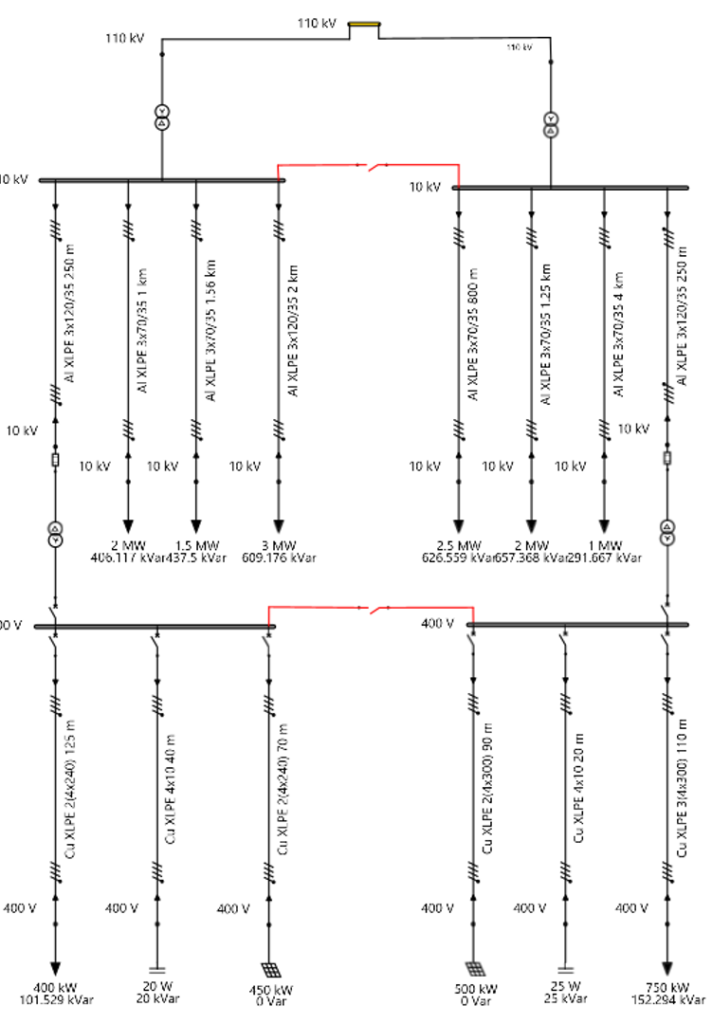## 2. General requirments

There are four main requirements that must be considered when connecting a renewable energy source to low voltage grid. They are as follow:

1. Voltage level (U, kV);
2. Voltage level difference from nominal grid voltage (dU, %);
3. Power flow;
4. Short circuit currents.

Voltage level; Voltage level difference from nominal grid voltage; Power flowTo ensure voltage quality in the electric network, certain standards must be maintained. For low voltage power grid its voltage limits must be within +10%/-10% (0.36-0.44 kV). According to the requirements voltage level and its difference must be evaluated when the energy source generation is at the highest capacity and when no loads are connected to the grid. Power flow test results with voltage level evaluations are shown in Figure 2. Disconnected loads are indicated with colour red.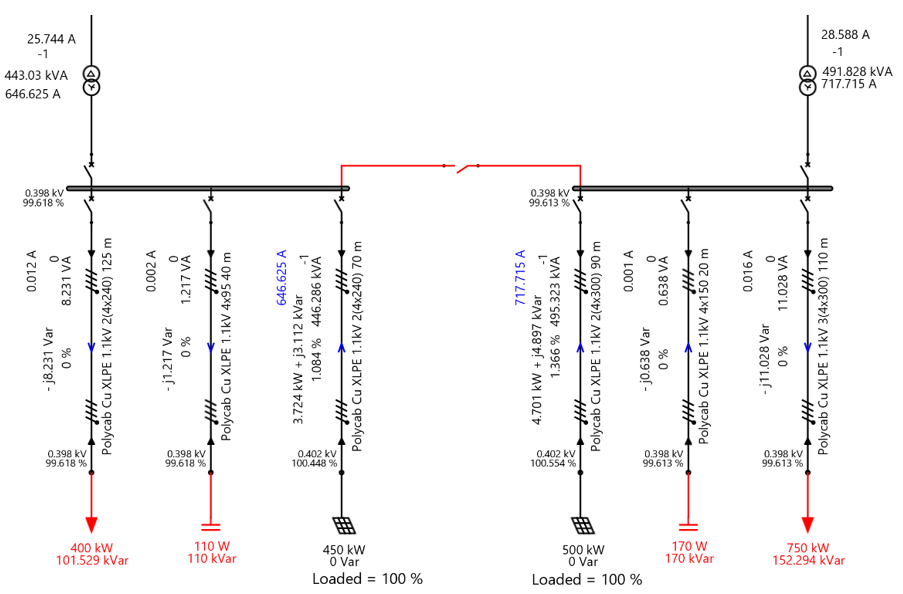## Figure 2 Power flow analysis (no faults in the grid)

It is also necessary to calculate power flows when there is a fault in the power grid or repairs are taking place. Figure 3 and Figure 4 show such situations when only one transformer is working, no loads are connected, and PV systems are generating the highest amount of energy.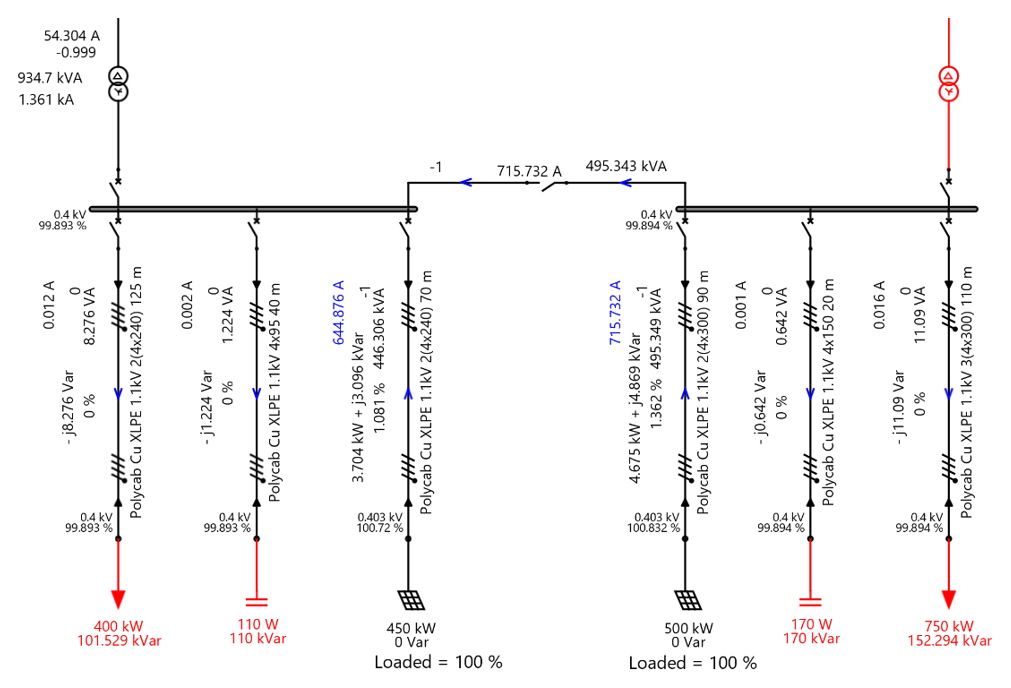## Figure 3 Power flow analysis (fault on the right side of the grid)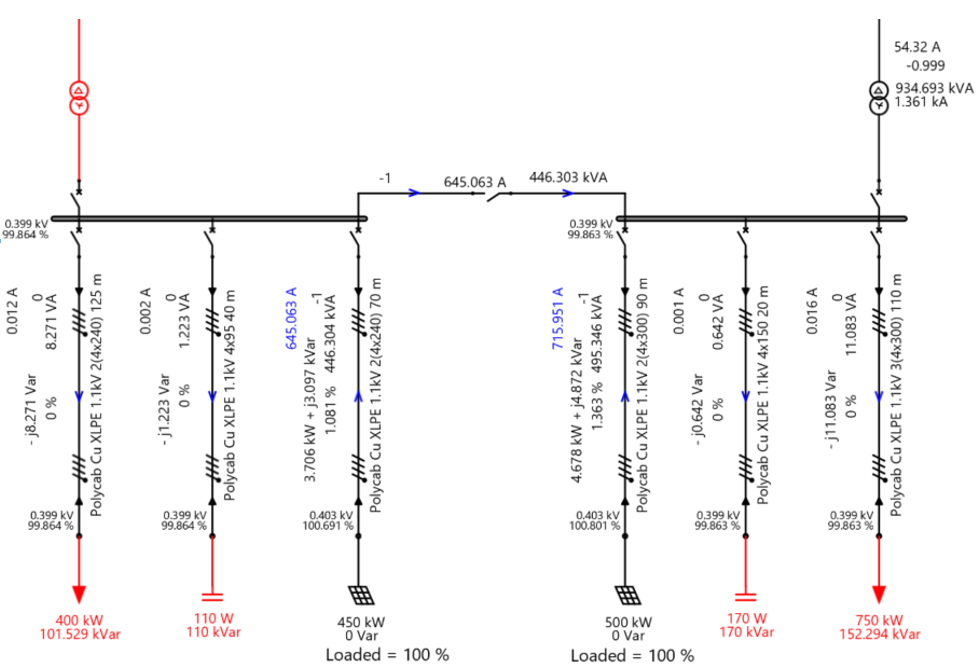## 2.1 Short circuit current analysis

According to the requirements it is necessary to evaluate three phase minimum and maximum short circuit currents as well as two phase minimum short circuit current. This was performed at the PV system’s connection point and near the inverter. Short circuit currents were calculated with no load condition, but these tests could also be carried out with loads by just clicking a few buttons if needed. Minimum short circuit current analysis near the inverter can be seen in Figure 5 and the maximum can be seen in Figure 6  The test at connection point can be found in Figure 7 (minimum) and Figure 8 (maximum).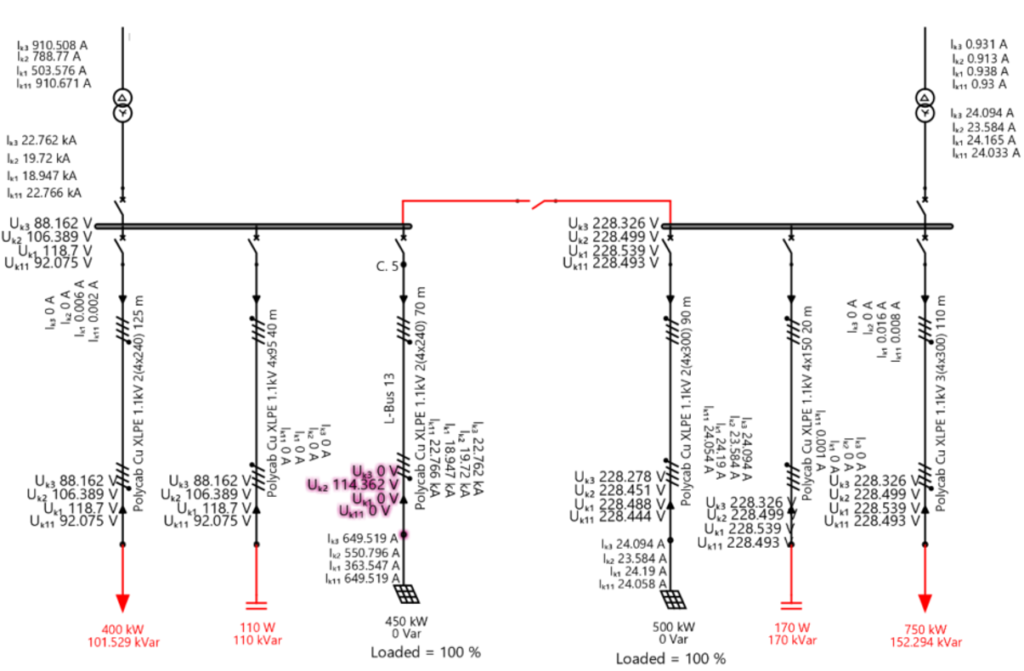## Figure 5 Minimum short circuit current analysis near the inverter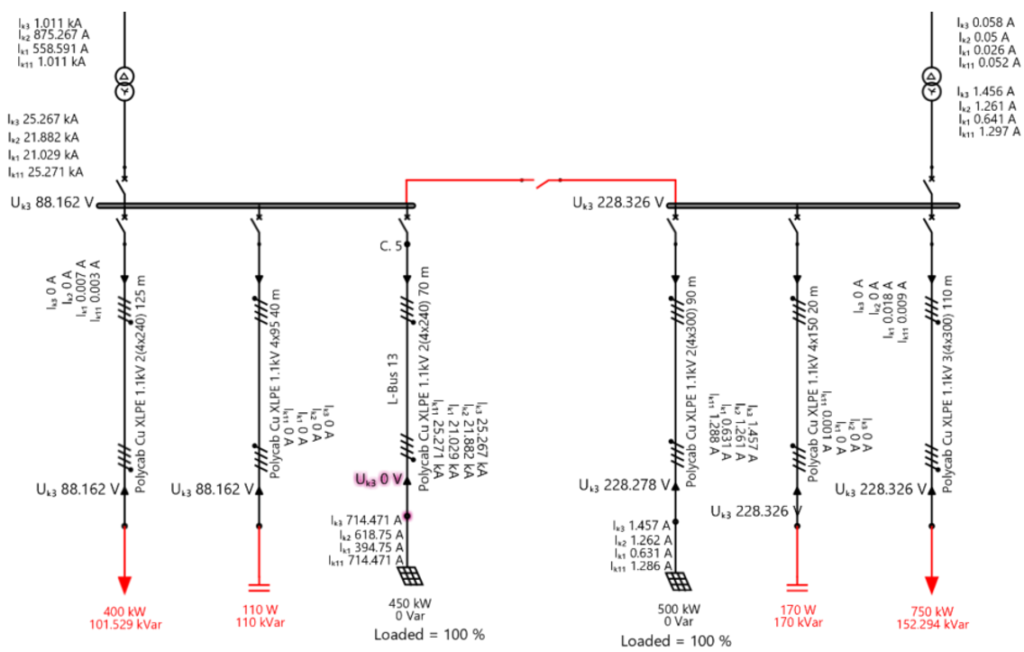## Figure 6 Maximum short circuit current analysis near the inverter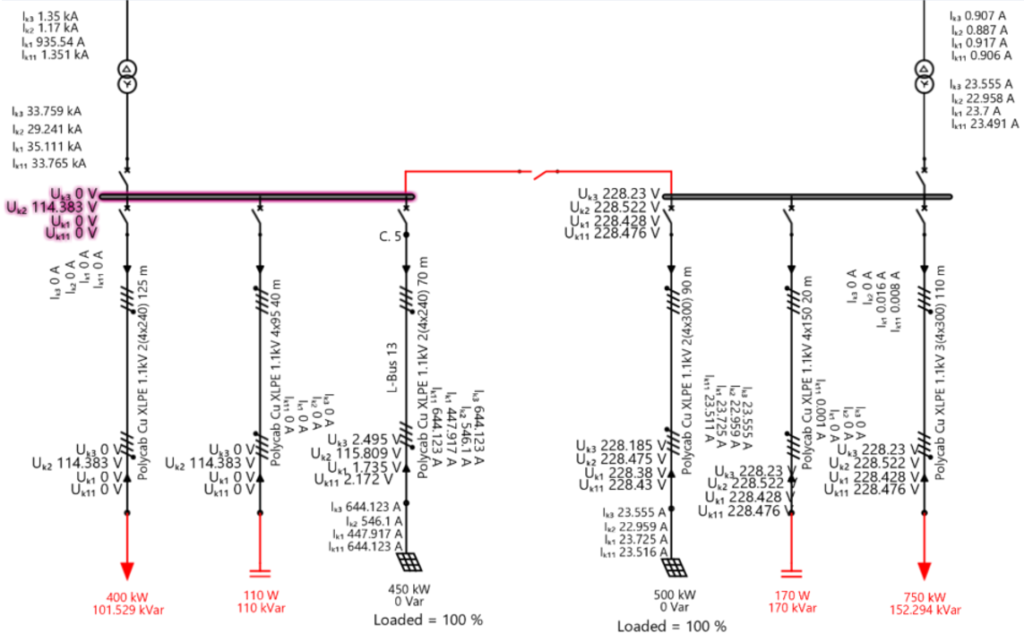## Figure 7 Minimum short circuit current analysis at connection point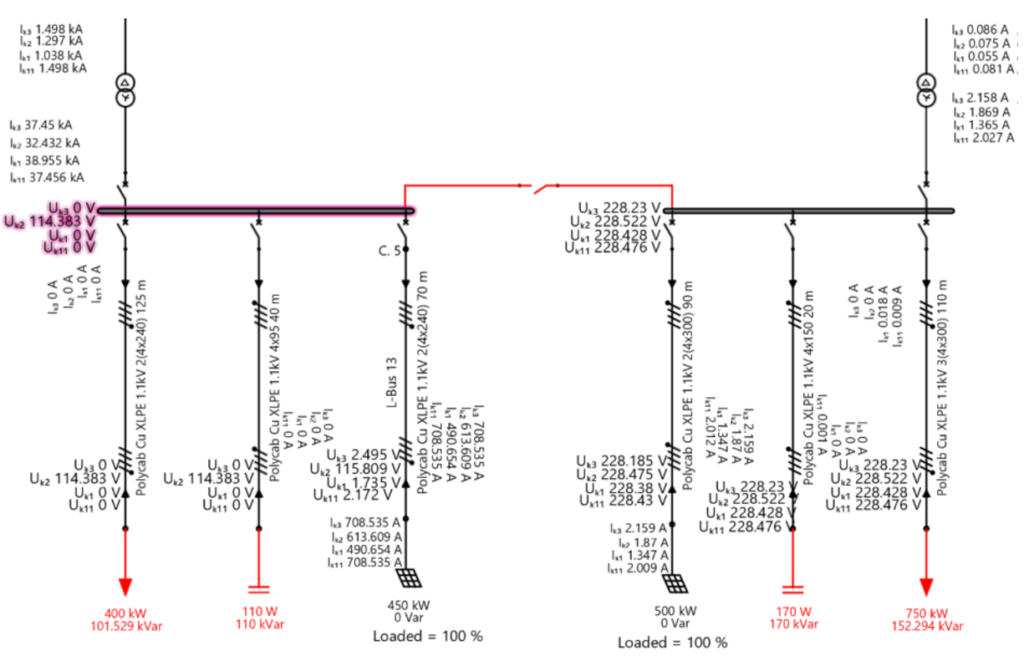## 3. Harmonic distortion analysis

Harmonic current distortions are another parameter that must be calculated when connecting a medium power level PV system. It must be calculated at the connection point and it cannot exceed 6.5% total harmonic distortion (THD). The analysis results can be seen in Figure 9 and Figure 10.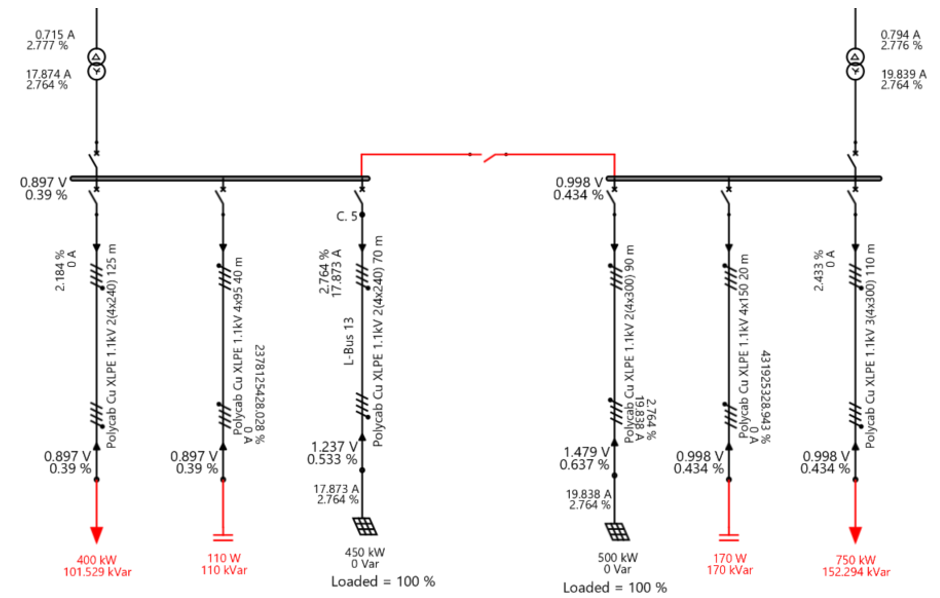## Figure 9 Total harmonic distortion at low voltage grid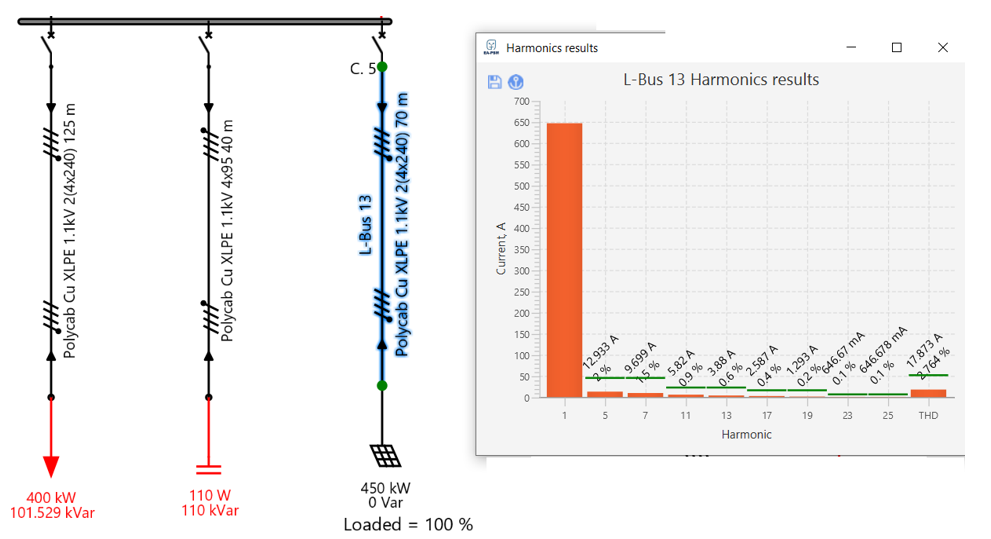## Figure 10 Total harmonic distortion at connection point

Figure 9 shows THD at different parts of the grid, current distortion can be seen on the lines and voltage distortion is visible near buses. It is required to calculate current THD at connection point, this can be found in Figure 10. If THD would exceed 6.5% a filter is needed to minimize the PV system’s created distortion.

Finally, voltage surge, short circuit phase angles and short circuit power at the point of connection to the grid must be calculated. Currently EA-PSM only counts short circuit phase angles, that is why the other two parameters will be calculated using appropriate formulas. Table 1 displays short circuit phase angle analysis results.

## Table 1 Short circuit current phase angle analysis results

 Analysed at Minimum or maximum short circuit current Short circuit current phase angle, ∠δ, ° PV system’s connection point to the grid Maximum -25.9 Minimum -27.9

Short circuit power at connection point is calculated by equation: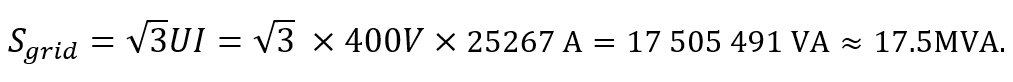Voltage surge at no load conditions is calculated by equation:## 5. Conclusion

Solar power plant numbers are growing, and it is important that PV systems do not disrupt the existing power grid. Voltage level must be within ±10% limits, total harmonic distortion for current at the connection point cannot exceed 6.5% and power flow calculation, short circuit current analysis, voltage surge, short circuit phase angles and short circuit power at the point of connection must be carried out when connecting a new medium power PV system to the grid. It is important to keep in mind that these requirements may be different in different countries.